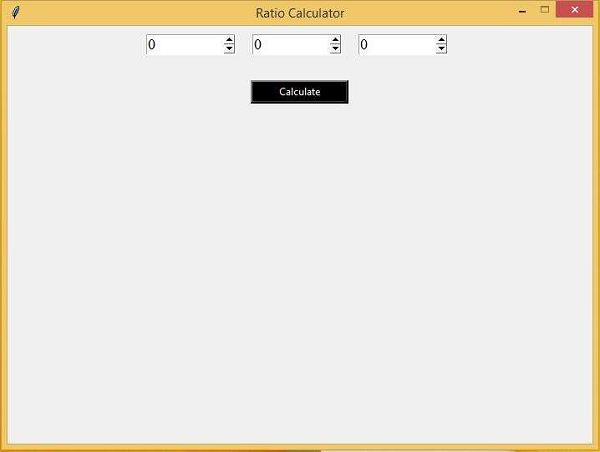# Ratio Calculator GUI using Tkinter

PythonTkinterGUI-Programming

In this article, we will see how to create a functional application that calculates the ratio. In order to make it fully functional, we will use SpinBox method that generally creates an ideal spinner for a value. This value can be modified using the spinner widget in the frame. Thus, a SpinBox object takes values in the range from minimum to maximum.

First, we will create a tkinter frame inside which we will define some widgets.

## Example

from tkinter import *
win = Tk()

win.title("Ratio Calculator")
win.geometry("600x500")
win.resizable(0,0)
#Create text Label for Ratio Calculator

label= Label(win, text="Ratio Calculator", font=('Times New Roman', 25))

#Define the function to calculate the value

def ratio_cal():
a1=int(a.get())
b1= int(b.get())
c1= int(c.get())

val= (b1*c1)/a1

x_val.config(text=val)

frame= Frame(win)
frame.pack()

#Create Spin Boxes for A B and C

a= Spinbox(frame, from_=0, to= 100000, font=('Times New Roman', 14), width=10)

b= Spinbox(frame,from_=0, to=100000, font=('Times New Roman', 14), width=10)

c= Spinbox(frame, from_=0, to=100000, font=('Times New Roman', 14), width= 10)

x_val= Label(frame, text="",font=('Times New Roman', 18))
x_val.pack(side=LEFT)

#Create a Button to calculate the result

Button(win, text= "Calculate",command=ratio_cal, borderwidth=3, fg="white",
win.mainloop()